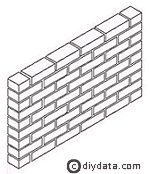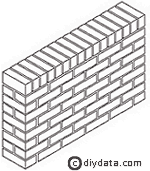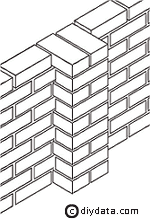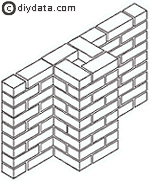home » general building » brick calculator

# Calculating bricks and blocks

One aspect of brickwork which often causes confusion is estimating how many bricks or blocks will be required for a planned wall - you don't want to run out before you finish but you also don't want a pallet load of material left unused when you have finished. Making these estimates is fairly straight forward using just a few figures.

First the number of bricks/blocks for the total wall is calculated, then the additional number of bricks for any piers. These are added together and then a 10% allowance should be added for wastage and breakages - the figures below do not include this allowance.

## Calculating the number of bricks

Standard, UK, metric bricks are roughly 215 x 102.5 x 65mm, the mortar joints used are normally about 10mm both horizontally and vertically.

### Half brick wallA half brick wide wall requires 60 bricks per square metre.

So the first stage is just to measure the height and length (including any piers) of the wall in metres, multiply them together to give the area in square metres, and then multiply this by 60.

So the total number of bricks for the wall is:

wall height (metres) x wall length (metres) x 60 = number of bricks

### One brick wallA one brick wide wall requires 120 bricks per square metre.

So the first stage is just to measure the height and length of the wall in metres, multiply them together to give the area in square metres, and then multiply this by 120.

So the total number of bricks for the wall is:

wall height (metres) x wall length (metres) x 120 = number of bricks

### Single brick piers in half brick wallsWhere single brick piers are built into a half brick wall, each pier requires an additional 14 bricks per vertical metre.

So the total number of bricks for the piers is:

number of piers x wall height (metres) x 14 = number of bricks

### One and a half brick piers in a half brick wallWhere one and a half brick piers are built into a half brick wall, each pier requires an additional 34 bricks per vertical metre.

So the total number of bricks for the piers is:

number of piers x wall height (metres) x 34 = number of bricks

## Calculating the number of blocks

Standard, UK, metric blocks are roughly 450 x 215 x 100mm (some are thicker but this will not effect the number of blocks required per square metre of wall), the mortar joints used are normally about 10mm both horizontally and vertically.

A single block wall requires 10 blocks per square metre.

So the total number of blocks for the wall is:

wall height (metres) x wall length (metres) x 10 = number of blocks